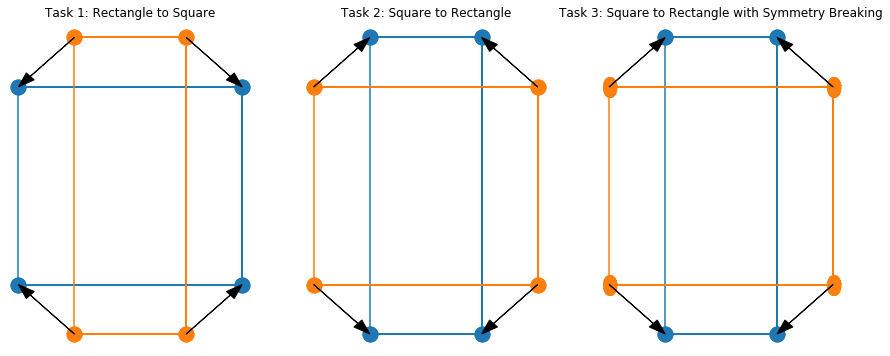## code by:¶@misc{mario_geiger_2019_3348277,
author       = {Mario Geiger and
Tess Smidt and
Wouter Boomsma and
Maurice Weiler and
Michał Tyszkiewicz and
Jes Frellsen and
Benjamin K. Miller},
title        = {mariogeiger/se3cnn: Point cloud support},
month        = jul,
year         = 2019,
doi          = {10.5281/zenodo.3348277},
url          = {https://doi.org/10.5281/zenodo.3348277}
}

There are some unintuitive consequences of using E(3) equivariant neural networks. The symmetry your output has to be equal to or higher than the symmetry of your input. The following 3 simple tasks are to help demonstrate this:

• Task 1: Distort a rectangle to a square.
• Task 2: Distort a square to a rectangle.
• Task 3: Distort a square to a rectangle -- with symmetry breaking.

We will see that we can quickly do Task 1, but not Task 2. Only by using symmetry breaking in Task 3 are we able to distort a square into a rectangle.

In :
import torch
from functools import partial
import numpy as np

import se3cnn
import se3cnn.SO3 as SO3
from se3cnn.point.operations import Convolution
from se3cnn.non_linearities import GatedBlock
from se3cnn.point.kernel import Kernel
from se3cnn.non_linearities import rescaled_act

import matplotlib.pyplot as plt
%matplotlib inline

from spherical import SphericalTensor

torch.set_default_dtype(torch.float64)

In :
# Define out geometry
square = torch.tensor(
[[0., 0., 0.], [1., 0., 0.], [1., 1., 0.], [0., 1., 0.]]
)
square -= square.mean(-2)
sx, sy = 0.5, 1.5
rectangle = square * torch.tensor([sx, sy, 0.])
rectangle -= rectangle.mean(-2)

N, _ = square.shape

markersize = 15

def plot_task(ax, start, finish, title, marker=None):
ax.plot(torch.cat([finish[:, 0], finish[:, 0]]),
torch.cat([finish[:, 1], finish[:, 1]]), 'o-', markersize=markersize)
ax.plot(torch.cat([start[:, 0], start[:, 0]]),
torch.cat([start[:, 1], start[:, 1]]), 'o-',
markersize=markersize + 5 if marker else markersize,
marker=marker if marker else 'o')
for i in range(N):
ax.arrow(start[i, 0], start[i, 1],
finish[i, 0] - start[i, 0],
finish[i, 1] - start[i, 1],

ax.set_title(title)
ax.set_axis_off()

fig, axes = plt.subplots(1, 3, figsize=(15, 6))
plot_task(axes, square, rectangle, "Task 3: Square to Rectangle with Symmetry Breaking", "$\u2B2E$")In these tasks, we want to move 4 points in one configuration to another configuration. The input to the network will be the initial geometry and features on that geometry. The output will be used to signify "displacement" of each point to the new configuration. We can represent displacement in a couple different ways. The simplest way is to represent a displacement as an L=1 vector, Rs=[(1, 1]]. However, to better illustrate the symmetry properties of the network, we instead are going to use a spherical harmonic signal or more specifically, the peak of the spherical harmonic signal, to signify the displacement of the original point.

First, we set up a very basic network that has the same representation list Rs = [(1, L) for L in range(5 + 1)] throughout the entire network. The input will be a spherical tensor with representation Rs and the output will also be a spherical tensor with representation Rs. We will interpret the output of the network as a spherical harmonic signal where the peak location will signify the desired displacement.

In :
class Network(torch.nn.Module):
super().__init__()
self.Rs = Rs
self.n_layers = n_layers
self.L_max = max(L for m,L in Rs)

sp = rescaled_act.Softplus(beta=5)

Rs_geo = [(1, l) for l in range(self.L_max + 1)]
Rs_centers = [(1, 0), (1, 1)]

number_of_basis=number_of_basis, h=100,

C = partial(Convolution, K)

self.layers = torch.nn.ModuleList([
GatedBlock(Rs, Rs, sp, rescaled_act.sigmoid, C)
for i in range(n_layers - 1)
])

self.layers.append(
Convolution(K, Rs, Rs)
)

def forward(self, input, geometry):
output = input
batch, N, _ = geometry.shape
for layer in self.layers:
output = layer(output.div(N ** 0.5), geometry)
return output


## Task 1: Distort a rectangle to square.¶

In this task, our input is a four points in the shape of a rectangle with simple scalars (1.0) at each point. The task is to learn to displace the points to form a (more symmetric) square.

In :
L_max = 5
Rs = [(1, L) for L in range(L_max + 1)]

model = Network(Rs)

params = model.parameters()
loss_fn = torch.nn.MSELoss()

In :
input = torch.zeros(1, N, sum(2 * L + 1 for L in range(L_max + 1)))
input[:, :, 0] = 1.  # batch, point, channel

displacements = square - rectangle
N, _ = displacements.shape
projections = torch.stack([SphericalTensor.from_geometry(displacements[i], L_max).signal for i in range(N)])

In :
iterations = 100
for i in range(iterations):
output = model(input, rectangle.unsqueeze(0))
loss = loss_fn(output, projections.unsqueeze(0))
if i % 10 == 0:
print(loss)
loss.backward()
optimizer.step()

tensor(0.0091, grad_fn=<MseLossBackward>)

In :
# Plot spherical harmonic projections
import plotly
from plotly.subplots import make_subplots
import plotly.graph_objects as go

In :
def plot_output(start, finish, output, start_label, finish_label):
rows, cols = 1, 1
specs = [[{'is_3d': True} for i in range(cols)]
for j in range(rows)]
fig = make_subplots(rows=rows, cols=cols, specs=specs)
fig.add_trace(go.Scatter3d(x=start[:, 0], y=start[:, 1], z=start[:, 2], mode="markers", name=start_label))
fig.add_trace(go.Scatter3d(x=finish[:, 0], y=finish[:, 1], z=finish[:, 2], mode="markers", name=finish_label))
for i in range(N):
trace = SphericalTensor(output[i].detach(), Rs).plot(center=start[i])
trace.showscale = False

output = model(input, rectangle.unsqueeze(0))